• 分享个大牛的人工智能教程。零基础！通俗易懂！风趣幽默！希望你也加入到人工智能的队伍来！请点击http://www.captainbed.net /* * Created by Chimomo * * 二叉搜索树的建树方法。简单的递归结构。 */ #...
分享一个大牛的人工智能教程。零基础！通俗易懂！风趣幽默！希望你也加入到人工智能的队伍中来！请点击http://www.captainbed.net

/*
* Created by Chimomo
*
* 二叉搜索树的建树方法。简单的递归结构。
*/

#include <iostream>

#define NULL 0

using namespace std;

template<class T>
struct BTNode {
T data;
BTNode<T> *pBTNodeLeft, *pBTNodeRight;

BTNode();

BTNode(const T &val, BTNode<T> *pBTNodeLeft = NULL, BTNode<T> *pBTNodeRight = NULL) {
this->data = val;
this->pBTNodeLeft = pBTNodeLeft;
this->pBTNodeRight = pBTNodeRight;
}

BTNode<T> *CopyTree() {
BTNode<T> *pBTNodeLeft, *pBTNodeRight, *pBTNode;
if (&data == NULL) {
return NULL;
}
pBTNodeLeft = pBTNodeLeft->CopyTree();
pBTNodeRight = pBTNodeRight->CopyTree();
pBTNode = new BTNode<T>(data, pBTNodeLeft, pBTNodeRight);
return pBTNode;
}
};

template<class T>
BTNode<T>::BTNode() {
pBTNodeLeft = pBTNodeRight = NULL;
}

template<class T>
void insertNode(BTNode<T> **pBTNode, T val) {
BTNode<T> *pBTNodeNew = (BTNode<T> *) malloc(sizeof(BTNode<T>));
pBTNodeNew->data = val;
pBTNodeNew->pBTNodeLeft = NULL;
pBTNodeNew->pBTNodeRight = NULL;

if (!(*pBTNode)) {
*pBTNode = pBTNodeNew;
} else if (pBTNodeNew->data < (*pBTNode)->data) {
insertNode(&(*pBTNode)->pBTNodeLeft, val);
} else {
insertNode(&(*pBTNode)->pBTNodeRight, val);
}
}

int main() {
int arr = {3, 1, 2, 6, 4, 5};
BTNode<int> *pBTNode = NULL;
for (int i = 0; i < 6; i++) {
insertNode(&pBTNode, arr[i]);
}
return 0;
}

// Output:
/*

*/


展开全文C++
• 查询一组数据的众数： 方法1：仅适用于一组数据只有一个众数的情况 1）首先对数据按照值的不同进行分组，并对每组的数据进行计数，再根据计数的大小进行降序排序； 2）上述1）结果集的第一行即要求取的众数...
查询一组数据的众数：

方法1：仅适用于一组数据只有一个众数的情况

1）首先对数据按照值的不同进行分组，并对每组中的数据进行计数，再根据计数的大小进行降序排序；

2）上述1）中结果集的第一行即要求取的众数所在的行。

方法2：适用于一组数据有一个或多个众数的情况

1）首先对数据按照值的不同进行分组，并对每组中的数据进行计数；

2）使用max函数找出统计个数的最大值及其对应的被统计值，此被统计值就是要求的众数。

创建测试用表：

# 创建学生成绩表：
id int,
name varchar(10),
score int (10)
)engine myisam charset utf8mb4;

# 向成绩表中插入数据：
(1,'张无忌',85),
(2,'李隆基',59),
(3,'王五',60),
(4,'曹操',79),
(5,'小明',90),
(6,'如花',60),
(7,'尉迟恭',100),
(8,'欧阳风',90),
(9,'刘备',90),
(10,'董永',99),
(11,'冯钰',83),
(12,'孙殿英',82);


查询学生成绩表garde中分数的众数及其出现的次数：

先查看garde表的整体信息：

select * from garde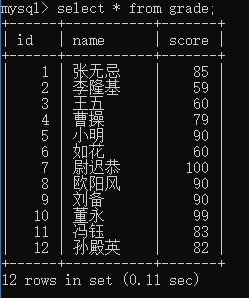方法1代码及查询结果：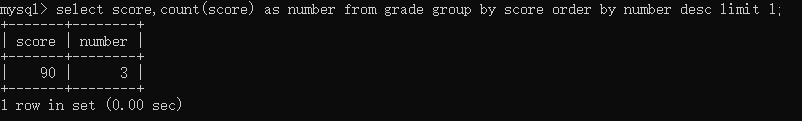方法2代码及查询结果：

为简化SQL语句，将方法2中的第一步处理——对数据按照值的不同进行分组，并对每组中的数据进行计数写成视图：

create view grouped_grade as
select score,count(score) as number from grade group by score;

select score,number from grouped_grade;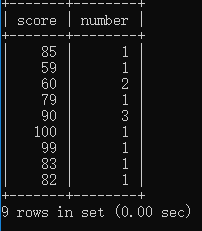select score,number from grouped_grade where number=(select max(number) from grouped_grade);

查询结果为：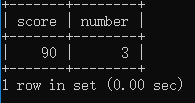查询一组数据的中位数：

网上看到一个很巧妙的方法：

SET @rowindex := -1;

SELECT
AVG(g.score) AS median
FROM
(SELECT @rowindex:=@rowindex + 1 AS rowindex,
score
ORDER BY score) AS g
WHERE
g.rowindex IN (FLOOR(@rowindex / 2) , CEIL(@rowindex / 2));

代码解析：上述查询方法的核心思想是对于一组要求其中位数的数据，无论数据的总行数是奇数还是偶数，都取这组数据排序后最中间的两个数的平均值作为中位数(当数据的总行数是奇数时，数据经排序后最中间的数取两次以计算平均值)。

上述代码的查询结果为：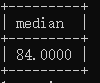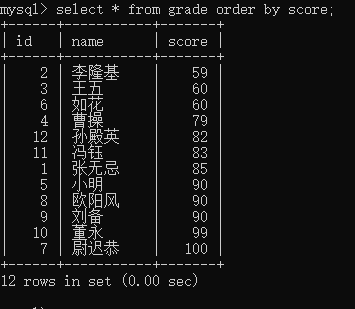经比较可知，前文查询出的中位数是正确的。

参考：

https://blog.csdn.net/qq_41080850/article/details/86310311

https://blog.csdn.net/hj7jay/article/details/78130419

展开全文MySQL
• 利用分治法求一组数据中最大的两个和最小的两个。 #define M 10 #include int a[]={3,6,0,7,8,20,16,24,10,18}; void maxtwo(int,int,int*,int*); void mintwo(int,int,int*,int*); void main() {...
利用分治法求一组数据中最大的两个数和最小的两个数。
#define M 10
#include<stdio.h>
int a[]={3,6,0,7,8,20,16,24,10,18};
void maxtwo(int,int,int*,int*);
void mintwo(int,int,int*,int*);
void main()
{      int max1,max2,min1,min2;
maxtwo(0,M-1,&max1,&max2);
printf("The max two elements:%d  %d\n",max1,max2);
mintwo(0,M-1,&min1,&min2);
printf("The min two elements:%d  %d\n",min1,min2);  }
void maxtwo(int i,int j,int *max1,int *max2)
{      int lmax1,lmax2,rmax1,rmax2;
int mid;
if(i==j) {*max1=a[i];*max2=a[i];}
else if (i==j-2)
{      if (a[i]>=a[i+1]&&a[i+1]>=a[i+2])
{*max1=a[i];    *max2=a[i+1];}
else if (a[i]>=a[i+2]&&a[i+2]>=a[i+1])
{*max1=a[i];    *max2=a[i+2];}
else if (a[i+1]>=a[i]&&a[i]>=a[i+2])
{*max1=a[i+1];  *max2=a[i];}
else if (a[i+1]>=a[i+2]&&a[i+2]>=a[i])
{*max1=a[i+1];  *max2=a[i+2];}
else if (a[i+2]>=a[i]&&a[i]>=a[i+1])
{*max1=a[i+2];    *max2=a[i];}
else{*max1=a[i+2];    *max2=a[i+1];}    }
else
{ mid=(i+j)/2;
maxtwo(i,mid,&lmax1,&lmax2);
maxtwo(mid+1,j,&rmax1,&rmax2);
if(lmax1>=rmax1)
{ *max1=lmax1;
if (lmax2>=rmax2)  *max2=lmax2;
else               *max2=rmax1;    }
else
{*max1=rmax1;
if (rmax2>=lmax2)  *max2=rmax2;
else               *max2=lmax1;   }    }   }
void mintwo(int i,int j,int* min1,int* min2)
{ int lmin1,lmin2,rmin1,rmin2;
int mid;
if(i==j) {*min1=a[i];*min2=a[i];}
else if(i==j-2)
{ if (a[i]<=a[i+1]&&a[i+1]<=a[i+2])
{*min1=a[i];    *min2=a[i+1];}
else if (a[i]<=a[i+2]&&a[i+2]<=a[i+1])
{*min1=a[i];    *min2=a[i+2];}
else if (a[i+1]<=a[i]&&a[i]<=a[i+2])
{*min1=a[i+1];  *min2=a[i];}
else if (a[i+1]<=a[i+2]&&a[i+2]<=a[i])
{*min1=a[i+1];  *min2=a[i+2];}
else if (a[i+2]<=a[i]&&a[i]<=a[i+1])
{*min1=a[i+2];   *min2=a[i];}
else{*min1=a[i+2];    *min2=a[i+1];}    }
else{ mid=(i+j)/2;
mintwo(i,mid,&lmin1,&lmin2);
mintwo(mid+1,j,&rmin1,&rmin2);
if(lmin1<=rmin1)
{*min1=lmin1;
if (lmin2<=rmin2)  *min2=lmin2;
else               *min2=rmin1;     }
else{*min1=rmin1;
if (rmin2<=lmin2)  *min2=rmin2;
else               *min2=lmin1;   }   }    }

展开全文• if(m==n)//分治子数组只有 { *min1=*min2=*max1=*max2=a[m]; } else//分治子数组不止 if(m==n-1)//分治子数组仅有2个 { if(a[m][n]) { *min1=a[m]; *min2=a[n]; *max1=a[n]; *max2=a[m...
基本步骤： 分解：将原问题分解为若干个规模较小、相互独立，与原问题形式相似的子问题；  解决：若子问题规模较小而容易被解决则直接解，否则再继续分解为更小的子问题，直到容易解决；  合并：将已求解的各个子问题的解，逐步合并为原问题的解。适用分治法策略的问题：可分解为k个独立求解的不同子问题，1＜k≤n；子问题与原问题结构相似，便于利用递归或循环；求出子问题的解之后，就可以推解出原问题的解。#include<stdio.h>
#define N 10

void max_min(int *a,int m,int n,int *min1,int *min2,int *max1,int *max2);

int main(void)
{
int a[N]={2,3,4,5,34,7,9,6,43,21};
int min1,min2;
int max1,max2;
max_min(a,0,N-1,&min1,&min2,&max1,&max2);
//这四个参数的大小关系为 min1<min2<max2<max1
printf("min1=%d min2=%d\n max1=%d max2=%d\n",min1,min2,max1,max2);
return 0;
}

void max_min(int *a,int m,int n,int *min1,int *min2,int *max1,int *max2)
{
int lmin1,lmin2,lmax1,lmax2;
int rmin1,rmin2,rmax1,rmax2;
int mid;

if(m==n)//分治子数组中只有一个数
{
*min1=*min2=*max1=*max2=a[m];
}

else//分治子数组中不止一个数
if(m==n-1)//分治子数组中仅有2个数
{
if(a[m]<a[n])
{
*min1=a[m];
*min2=a[n];
*max1=a[n];
*max2=a[m];
}
else
{
*min1=a[n];
*min2=a[m];
*max1=a[m];
*max2=a[n];
}
}

else//分治子数组中有超过2个数
{
mid=(m+n)/2;
max_min(a,m,mid,&lmin1,&lmin2,&lmax1,&lmax2);
max_min(a,mid+1,n,&rmin1,&rmin2,&rmax1,&rmax2);

//**************************************************
//确定出数组中最小的两个数
//**************************************************
if(lmin1<rmin1)//左子数组最小数<右子数组最小数
{
if(lmin2<rmin1)
{
*min1=lmin1;
*min2=lmin2;
}
else
{
*min1=lmin1;
*min2=rmin1;
}
}
else//右子数组最小数<左子数组最小数
if(rmin2<lmin1)
{
*min1=rmin1;
*min2=rmin2;
}
else
{
*min1=rmin1;
*min2=lmin1;
}

//**************************************************
//确定出数组中最大的两个数
//**************************************************
if(lmax1>rmax1)//左子数组最大数>右子数组最大数
{
if(lmax2>rmax1)
{
*max1=lmax1;
*max2=lmax2;
}
else
{
*max1=lmax1;
*max2=rmax1;
}
}
else//右子数组最大数>左子数组最大数
if(rmax2>lmax1)
{
*max1=rmax1;
*max2=rmax2;
}
else
{
*max1=rmax1;
*max2=lmax1;
}
}
}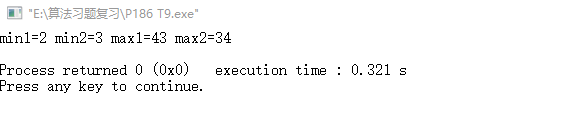展开全文• #include&lt;iostream&gt; #include&...//创建个结构体，表示链表的节点类型  struct node{  int data;  struct node *next; }; int main() {  struct node *head,*p,*q,*t;  int n,a...链表
• 众数是指在一组数据中，出现次数最多的。例如：1, 1, 3 出现次数最多的为 1，则众数为 1。 给定一组，你能求出众数吗？ Input 输入数据有多组（数据组不超过 50），到 EOF 结束。 对于每组数据： •...c++
• 1.有一组正整数数据，找出其中的质数及其个，并求出数据中质数的和。要求用函数is_prime(x)实现质素判断，可考虑用函数prime_sum()实现质素求和处理，不做要求。（30分） 例如：假设正整数数据为：56,41,70,31,83...
• 1.引言 我们在生活非常需要去比较两组数据是不是一样。比如说你对你的客户进行了两次...我们需要找出第一组中与第二组的不一样的数据。方法如下。 方法： 用这个 =IF(COUNTIFS(A:A, E,B:B, F,C:C, G)>0,"","不excel Excel EXCEL office windows
• 或许当你看到行代码的...）就像进行数据处理的时候，有时会遇到求极值（最大值、最小值）、平均值、和四分位数（25%、 75%）的情况。 这篇博客就是你的福音，让你绝对0基础使用python 进行数据分析。 ...
• 如题，在面试和实际应用，经常会碰到一个场景：读入以指定符号间隔的一组数据，放入数组当中。 看了不少博客，总结了一个个人目前觉得比较简便的方法（其实和java比也一点不简便。。。。） 基本思路就是：将输入...c++学习
• /// 获得一组数据中的个数，最大值，最小值，平均值，标准差 /// /// 一组数据 /// 个，最大值，最小值，平均值，标准差 public double[] GetStatisData(string sData) { double[] dResult = new ...string
•期望方差 峰度系数 变异系数
• 一组数中的最大值和最小值，例如我们有五个数字     int ary;  int max;  int min;  printf("请输入数组中的五个数字：\n");  for (int i=0; i5; i++)  {  scanf("%d",&ary[i]); //...c语言
• 设有一组N个而要确定...下面我们使用JAVA语言来编程找出一组数据中的最大值。  public class SelectMaxNum { /** * @param args * 找出一组数据中的最大值或者最小值 */ public static void main(Stringjava 算法 最大值 最小值
• 不论是 matlab 还是 R 语言，根据一组数据拟合分布函数时，只能拟合出特定分布的参数值，并不能给出最适合这组数据的分布函数。例如，matlab 有 normfit 函数来计算一组数据拟合成正态分布时的均值和方差，wblfit ...
• 查找一组数中的最大、最小值 /** * 查找一组数中的最大数 * @param nums 数组指针 * @param step sizeof(type) * @param n 该组数中有几个数 * @return 未找到返回-1，找到返回该数的索引 */ int findMax...C语言 最大值最小值 查找算法
• matlab画一组数据的频率分布图 L空间度汇总.xlsx B=xlsread(‘L空间度汇总.xlsx’); %注意点，待处理数据的excel文件要和脚本文件路径相同，即放置在同一文件夹下，否则无法正确读入数据。 m_1=0; m_2=0; m_3=...matlab
• 如何生成一组数据元素随机排列【随机打乱顺序，产生新的排列】 1. 使用的matlab函数： randperm() 　1)语法格式：R = randperm(n)　2)函数功能：将数字1:n进行随机排列，排列结果存储在行向量内　3)EX: 输入...
• import java.util.Scanner;public class HomeWork02 { public static void main(String[] args) { Scanner sc = new Scanner(System.in);... //先接收一组数字例如3216554684651454644 int [] arr =...习题
• 最近在看机器学习的东西时发现了一些特别好玩的东西，机器学习又分为训练集和测试集，如何把一组很大的数据分为这两个集合呢？可以使用接下来的函数完成： 当然由于random这个随机数生成函数每次产生的不...机器学习
• 在很多模型及假设检验中都需要满足个假设条件：数据需服从正态分布。这篇文章主要讲讲如何判断数据是否符合正态分布。主要分为两种方法：描述统计方法和统计检验方法。 描述统计方法 描述统计就是用描述的数字或...python 统计学
• 快速排序法：通过趟排序将要排序的数据分割成独立的两部分，其中部分的所有数据都比另部分的所有数据要小，然后再按此方法对这两部分数据分别进行快速排序（此过程可以用到函数递归的方法）。 #include #...
• 数组给定十个数字，首先让第是最大的，与之后的进行比较，得到下个最大的，在与下比较。 #include &lt;stdio.h&gt; int main() { int a = { 5,9,8,7,15,20,11,44,23,4 }; int i...
•  如果是一组数据，要绘制它的直方图，这个时候，我们既没有这组数据的唯一值，又没有唯一值出现过的次数。那我们首先就需要得到这些唯一值，以及他们出现的次数。  如果是小数据样本(w级以下)，那么不用担心，你...
• c表a表查询语句select a.*,c.* FROM a LEFT JOIN c on FIND_IN_SET(a.a_id,c.a_id)
• 输入一组5个float类型的数据，要求输出这组数据，并且输出这组数据的平均值 最大值 最小值。 格式： 输入第 1 数据: 45 输入第 2 数据: 55 输入第 3 数据: 60 输入第 4 数据: 65 输入第 5 数据: 70 这组数据为:45.0 ...
• 最近一个项目的开发遇到一个需求，有一组数1,2,3,4,5,6,7,8,0.如何将一组随机打乱随机生成比如0-9的任意排列的一组数。比如生成0.6.1.2.3.4.5.7.8等等。 算法思路： 1、使用Arraylist存放这组 2、随机生成...
• #include&lt;stdio.h&gt;int main(){ int i,j,n,a... //n表示这组数据有n个。 scanf("%d",&amp;n); for(i=0;i&lt;n;i++) scanf("%d",&amp;a[i]); i=0; j=n-1; whil...C...